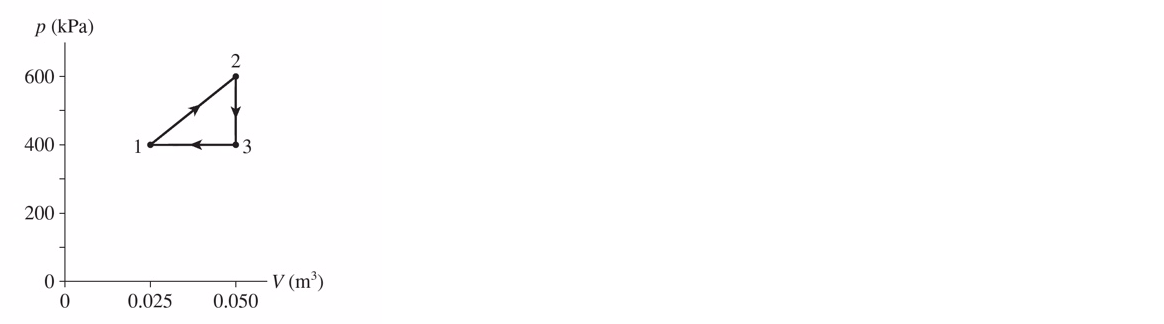# Problem: An engine uses 1.4 mol of a monoatomic ideal gas undergoing the cycle shown in the following figure. a) Determine T1, T2, and T3.b) Make a table showing ΔU, W, and Q for each step of the cycle. c) What is the engine's thermal efficiency?

###### Problem Details

An engine uses 1.4 mol of a monoatomic ideal gas undergoing the cycle shown in the following figure.

a) Determine T1, T2, and T3.

b) Make a table showing ΔU, W, and Q for each step of the cycle.

c) What is the engine's thermal efficiency?